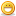1. ## Vector-class with template

Okay, this is actually C++ but i didnt want to open a new thread for it

basically i wrote a simple 3d vector class and i wanted to template it in the way you can pass in any 3d vector in its constructor:

PHP Code:
``` template< typename T >class Vec3{    public:        T x , y , z;        template< class Tvec >        Vec3( const Tvec& v );};template< typename T >template< class Tvec >Vec3< T >::Vec3( const Tvec& v ){    x = T( v.x );    y = T( v.y );    z = T( v.z );}//Needed useage (Bullet's btVector3 as example):btVector3 v1 = btVector3( 1.0 , 2.0 , 3.0 );Vec3 v2 = Vec3( v1 );  ```
The error:
Code:
```error C2228: left of '.x' must have class/struct/union
error C2228: left of '.y' must have class/struct/union
error C2228: left of '.z' must have class/struct/union```Reply With Quote

2. Thank you Kungi did some more testing and changed the function from the constructor to a normal method called 'from' - voilà the error is gone
I dont know if its done right or if i did something on the constructor wrong...mhhh actually i would like to work with the constructor on there^^Reply With Quote

3. PHP Code:
``` template< typename T > class Vec3 {     public:         T x , y , z;         //explicit to stop unlimited implicit typeconersations a la Vec3<int> v = randomType;         //you have declare it this way: Vec3<int> v( randomType );         template< class Tvec >         explicit Vec3( const Tvec& v ); }; template< typename T > template< class Tvec > Vec3< T >::Vec3( const Tvec& v ) {     this->x = T( v.x );     this->y = T( v.y );     this->z = T( v.z ); }  ```
seems to work

EDIT:
seems that my first example now compiles fine also.. ;(

@kung under me: heheheh we will see, give me some time xxPReply With Quote

4. Not even 20 lines and nobody understands C++ anymore, C FTW :>Reply With Quote

####Posting Permissions

• You may not post new threads
• You may not post replies
• You may not post attachments
• You may not edit your posts
•